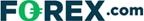# Analysis Of Fibonnaci Retracement: Uses & What It Tells Traders

Illustrated Introduction to Fibonacci Retracement
Last Updated:

In this Fibonacci technical analysis guide, we focus on Fibonacci retracement as a trading tool.

Read on to learn what Fibonacci retracement is, how it occurs on trading chars, and why people continue to use this old mathematical formula to trade strategically.

We also distinguish the difference between Fibonacci retracements from Fibonacci extensions.

## What Is Fibonacci Retracement?

Fibonacci trading tools utilize special ratios that naturally occur in nature to help predict points of support or resistance.

Fibonacci numbers are 1, 1, 2, 3, 5, 8, 13, 21, 34, 55, 89, etc. The sequence occurs by adding the previous two numbers (i.e. 1+1=2, 2+3=5)

The main ratio used is .618, this is found by dividing one Fibonacci number into the next in sequence Fibonacci number (55/89=0.618).

### Speculation On Naturally Recurring Patterns

The logic most often used by Fibonacci based traders is that since Fibonacci numbers occur in nature and the stock, futures, and currency markets are creations of nature – humans.

Therefore, the Fibonacci sequence should apply to the financial markets.

There are many other Fibonacci tools used by traders, they include:

## How To Calculate Fibonacci Retracement Levels

Arguably the most heavily used Fibonacci tool is the Fibonacci Retracement. To calculate the Fibonacci Retracement levels, a significant low to a significant high should be found.

From there, prices should retrace the initial difference (low to high or high to low) by a ratio of the Fibonacci sequence, generally the 23.6%, 38.2%, 50%, 61.8%, or the 76.4% retracement.

### A Demonstration Of Retracement Levels

For the examples of this section, the S&P 500 Depository Receipts (SPY) will be used based on the logic that the S&P 500 is a broad measure of human nature, thus the Fibonacci sequence should apply very well.

Nevertheless, the Fibonacci sequence is applied to individual stocks, commodities, and forex currency pairs quite regularly.

The chart above shows the 38.2% retracement acting as support for prices.

### How Is The Trendline Drawn?

Note that a trendline was drawn from a significant low (beginning of trend) to a significant high (end of trend); the trading software calculated the retracement levels.

The chart below of the SPY’s shows that Fibonacci Retracements can be used to retrace downtrend moves as well:

Notice after the bottom in the S&P 500, that price rallied to the 23.6% retracement level and then was promptly rejected downwards.

### How It This Show In Price Movements?

After breaking resistance a few months later, the 23.6% retracement became support (see: Support & Resistance). The price rallied up to the 50% retracement level, where it ran up against resistance.

Price continued to fluctuate between the 38.2% retracement level (acting as support) and the 50% retracement level (acting as resistance).

There are many other Fibonacci tools like Fibonacci Arcs available to stock, forex, options, CFD, or futures traders.

## Where Can I Start Trading And Use Fibonacci Retracement?

If you are interested in trading using technical analysis, have a look at our reviews of these regulated brokers available in to learn which charting & analysis tools they offer:

CFDs are complex instruments and come with a high risk of losing money rapidly due to leverage. Between 74%-89% of retail investor accounts lose money when trading CFDs. You should consider whether you can afford to take the high risk of losing your money.

Learn more about technical analysis indicators, concepts, and strategies including Momentum, Elliot Waves, Market Thrust, and understanding Moving Averages.

Also see our guides on Forex, Crypto and Option brokers to find out which tools brokerages offer their clients.

## FAQs

### What are the Fibonacci retracement levels in trading?

The Fibonacci retracement levels are 23.6%, 38.2%, 61.8%, and 78.6%. Traders also use 50% as a retracement ratio. The 50% mark is used as a mid-point between two price positions considered significant. Then, traders can create new retracement levels to determine possible support and resistance price points.

### What is the difference between Fibonacci retracement and extension?

The primary difference between Fibonacci retracements and Fibonacci extensions as trading tools is that extensions are typically used to determine when to cash out from a trade. Retracements, on the other hand, are used to determine a desirable entry point, although they can also be used to plan an exit strategy.

### Plus500 is not available in the US

Legitimate CFD brokers, like Plus500, cannot accept US clients by law## US traders welcome at these brokers:

### Cryoptocurrencies:• Trade 14+ major crypto coins
• Includes Bitcoin, Ethereum & Ripple
• Super simple setup